Home / Electrical / SSC Junior Engineer Exam Paper 2018 Shift-9 (Electrical Engineering)

# SSC Junior Engineer Exam Paper 2018 Shift-9 (Electrical Engineering)

SSC je electrical
#jeelectrical #sscjeelectrical

SSC Junior Engineer Exam Paper – 2018 “held on 27 January 2018” Morning Shift (Electrical Engineering)

Q 1: Why are same types of cells connected in parallel?
Options:
1) To decrease the voltage rating
2) To increase the voltage rating
3) To decrease the current rating
4) To increase the current rating
Answer: To increase the voltage rating

Q 2: There are N resistances, each are connected in parallel having value R with equivalent resistance of X. What will be the total resistance when these N resistances are connected in series?
Options:
1) NX
2) RNX
3) X / N
4) N2X

Q 3: Which of the following is equivalent to 0.5 kWh?
Options:
1) 1800000 W
2) 1800000 J
3) 18000000 J
4) 36000000 J

Q 4: What is the conductivity (in Mhos/m) of a 2 Ohm circular wire, when the length and the diameter of the wire are 10 m and 0.8 m respectively?
Options:
1) 10
2) 1
3) 0.1
4) 5

Q 5: ‘Erg’ is a unit of measurement for ________.
Options:
1) Energy
2) Power
3) Voltage
4) Impedance

Q 6: What is the equivalent capacitance (in μF) between the terminals A and B in the circuit given below ?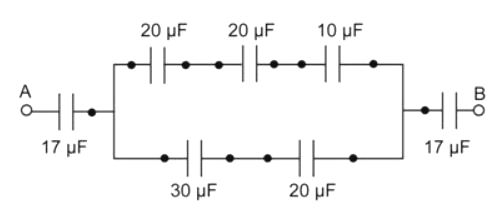Options:
1) 4.56

2) 5.67
3) 18.58
4) 51

Q 7: What is the equivalent inductance (in H) between the terminals A and B in the circuit given below ?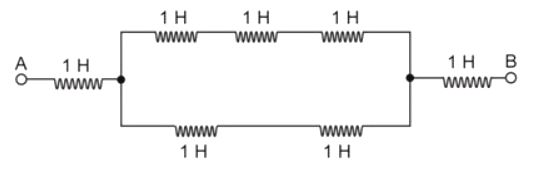Options:
1) 1

2) 1.42
3) 3.2
4) 7

Q 8: Which of the following quantity will remain the same, when a layer of Teflon is inserted between the plates of a charged parallel plate capacitor?
Options:
1) Capacitance
2) Charge
3) Energy of the capacitor
4) Potential

Q 9: What will be the value of resistance (in kilo-ohms) of a carbon composition resistor having color-coding of brown-black-brown-black?
Options:
1) 400
2) 200
3) 300
4) 100

Q 10: Which of the following is NOT a type of capacitor?
Options:
1) Ceramic
2) Electrolytic
3) Film
4) Wire wound

Q 11: Which of the following is TRUE in case of Substitution theorem?
Options:
1) The initial condition of the rest of the circuit changes, if a network element is replaced by a voltage source having an equal voltage as the voltage across the element at every instant of time.
2) The initial condition of the rest of the circuit changes, if a network element is replaced by a voltage source having an equal current as the voltage across the element at every instant of time.
3) The initial condition of the rest of the circuit remains same, if a network element is replaced by a voltage source having an equal voltage as the voltage across the element at every instant of time.
4) The initial condition of the rest of the circuit changes, if a network element is replaced by a voltage source having an equal voltage as the current across the element at every instant of time.
Answer: The initial condition of the rest of the circuit remains same, if a network element is replaced by a voltage source having an equal voltage as the voltage across the element at every instant of time.

Q 12: Which of the following theorem states that the sum of instantaneous power in ‘n’ number of branches of an electrical network is zero?
Options:
1) Compensation
2) Maximum power transfer
3) Superposition
4) Tellegen’s

Q 13: Kirchhoff’s voltage law is based on which of the following principle?
Options:
1) Conservation of charge
2) Conservation of energy
3) Conservation of force
4) Conservation of momentum
Answer: Conservation of energy

Q 14: What is the value of an unknown voltage “V” (in V) across the terminal A and B, in the circuit given below ?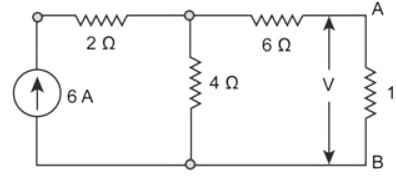Options:
1) 6

2) 10
3) 12
4) 16

Q 15: What will be the value of source impedance (in Ohms) for transmitting maximum power to the load in the circuit given below ?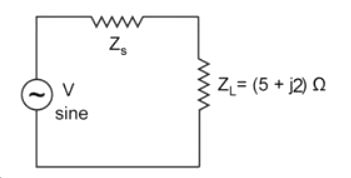Options:
1) 5 + j 2

2) 5 – j 2
3) 2 + j 5
4) 2 – j 5
Answer: 5 – j 2

Q 16: Determine the value of power dissipated (in W) through the 20 Ω resistor of the circuit given below.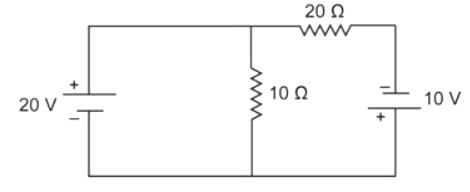Options:
1) 30

2) 45
3) 60
4) 75

Q 17: Determine the power dissipated (in W) by the 25 Ω resistor in the circuit given below.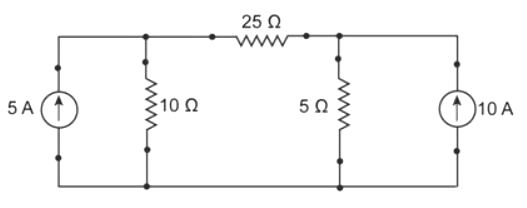Options:
1) 0

2) 25
3) 125
4) 156.25

Q 18: Determine the total current ‘I’ (in A) supplied by the voltage source in the circuit given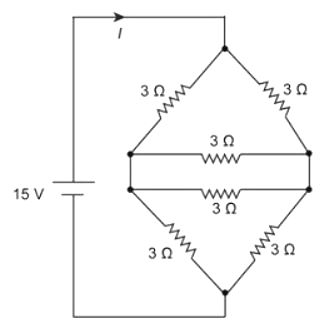Options:
1) 3
2) 4
3) 5
4) 6

Q 19: Determine the maximum power (in W) transferred from the source to the load of the circuit given below.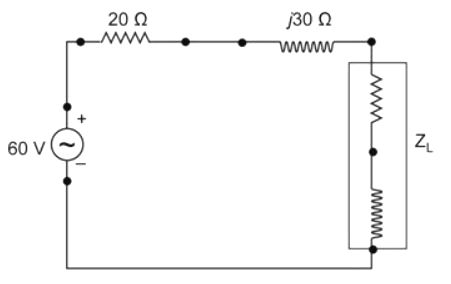Options:
1) 18
2) 30
3) 45
4) 90

Q 20: Determine the Norton’s current (in A) and Norton’s resistance (in Ω) respectively, for the given electrical circuit.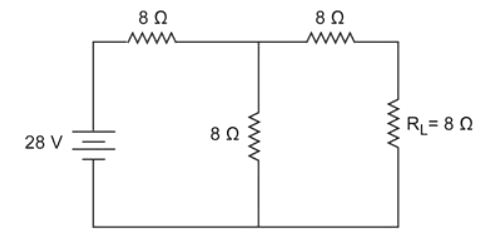Options:
1) 1.167, 12
2) 2.345, 14
3) 4.434, 16
4) 2.346, 10

Q 21: The S.I unit of electric charge is ______.
Options:
1) Henry
2) Coulomb
3) Tesla
4) Weber

Q 22: Which of the following is a diamagnetic material?
Options:
1) Aluminium
2) Oxygen
4) Nickel

Q 23: Which of the following material shows paramagnetism?
Options:
1) Copper
2) Iron
3) Titanium
4) Silver

Q 24: Which of the following is the CORRECT expression for Gauss’ Law?
Options:
1) φϵ = q / ϵ0
2) φϵ = ϵ0 / q
3) φϵ = 4E / H
4) φϵ = 4H / E

Q 25: Determine the intensity of magnetization (in A/m) of a magnet, when its pole strength is 100 Wb and has a pole area of 70 sq. m.
Options:
1) 4.98
2) 3.65
3) 2.53
4) 1.43
[sociallocker]
Q 26: Hysteresis loss occurring in a material does NOT depend on which of the following parameters?
Options:
1) Hysteresis constant
2) Magnetic flux density
3) Frequency
4) Reluctivity

Q 27: Determine the eddy current loss (in W) in a material having eddy current coefficient of 1, thickness of 0.02 m and a volume of 1 cubic metre, which is kept in a magnetic field of maximum flux density of 2 T and supplied by a frequency of 50 Hz.
Options:
1) 2
2) 3
3) 4
4) 5

Q 28: What will be the self-inductance (in mH) of a 4 m long air-core solenoid, if the diameter of the solenoid is 50 cm and has 300 turns?
Options:
1) 5.54
2) 6.94
3) 7.85
4) 8.64

Q 29: What is the coupling factor between the two coils, each having self inductance of 25 mH and the mutual inductance between the two is 25 mH?
Options:
1) 0.5
2) 0.75
3) 1
4) 2

Q 30: Determine the magnitude of the EMF (in V) induced between the axis of rotation and the rim of the disc, when the disc of radius 10 cm rotates with an angular velocity of 10 revolutions per second, which is placed in a magnetic field of 4 T acting parallel to the rotation of the disc.
Options:
1) 0.5
2) 0.75
3) 1
4) 1.25

Q 31: Determine the peak value (in A) of the current used by a motor rated at 220 V, 30 A.
Options:
1) 21.2
2) 30
3) 42.4
4) 51.9

Q 32: What will be the frequency (in Hz) of a sinusoidal wave, when the time period is 20 ms?
Options:
1) 50
2) 60
3) 40
4) 30

Q 33: Determine the instantaneous value of the alternating current (in A), Which is represented by i (t) = 10 sin (25t – 15)A, if the value of t is 3 ?
Options:
1) 9.36

2) 7.87
3) 8.66
4) 4.42

Q 34: At resonant frequency, the impedance of a series resonant circuit is _______________.
Options:
1) zero
2) maximum
3) infinite
4) minimum

Q 35: Determine the value of the phase angle (in degrees) in a series RC circuit having a resistance of 20 ohms and capacitive reactance of 11.56 ohms when supplied by a frequency of 50 Hz.
Options:
1) 60
2) 30
3) 90
4) 45

Q 36: A 3-phase delta connected system is supplied by a line voltage of 200 V. The value of phase current is 30 A. What is the total power consumed (in W) by the system, if the current lags the voltage by 30 degrees?
Options:
1) 16.32
2) 14.45
3) 15.59
4) 16.69

Q 37: What will be the capacitive susceptance (in Siemens) of the circuit given below ?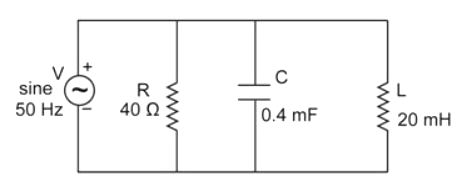Options:
1) 0.126

2) 1.26
3) 12.6
4) 126.1

Q 38: Determine the reactive power (in kVAR) of a 3-phase delta connected system having a line voltage of 400 V, line current of 40 A and the phase difference between the voltage and current is 45 degrees.
Options:
1) 14.4
2) 15.8
3) 18.7
4) 19.6

Q 39: Determine the quality factor of a series RLC circuit, when the value of capacitance is 0.02 mF, the value of inductance is 8 mH and the value of resistance is 40 ohms.
Options:
1) 0.5
2) 0.4
3) 0.8
4) 1.3

Q 40: What will be the time (in seconds) taken by the inductor reach its maximum steady state value in a series RL circuit, when the value of the inductance is 0.8 H and the value of the resistance is 10 Ohms?
Options:
1) 0.2
2) 0.6
3) 0.4
4) 0.8

Q 41: Which one of the following is the dimension of energy?
Options:
1) ML2 / T3
2) ML2 / T2
3) T2 / ML2
4) ML2 / QT2
Q 42: Which one of the following statement is NOT TRUE about the MI type instruments?
Options:
1) MI type Instruments are suitable for both AC and DC circuits.
2) Frictional error in MI type instruments is very less.
3) The torque weight ratio of MI type instruments is high.
4) The instrument cost is much higher as compared to PMMC type instruments.
Answer: The instrument cost is much higher as compared to PMMC type instruments.

Q 43: Which one of the following statement is NOT TRUE about multimeter?
Options:
1) Multimeter can be used for the measurement of voltage.
2) Multimeter can be used for the measurement of power.
3) Multimeter can be used for the measurement of resistance.
4) Multimeter can be used for the measurement of current.
Answer: Multimeter can be used for the measurement of power.

Q 44: Which one of the following is the main cause of magnetic decay in PMMC type instrument?
Options:
1) Variation in the resistance of the moving coil
2) Quality of spring
3) Aging of the spring
4) Aging of the magnets
Answer: Aging of the magnets

Q 45: Determine the quality factor in Hay’s bridge given below, if the bridge is supplied by a frequency of 50 Hz.

Options:
1) 2

2) 1
3) 0
4) 4

Q 46: Determine the apparent power (in W) of a circuit, if the circuit have a power factor of 0.8 and the reactive power of the circuit is 60 W.
Options:
1) 80
2) 75
3) 60
4) 55

Q 48: A building has 3 floors and each floor has 4 fans of 50 W that operates for 12 hours a day and one air conditioner of 3000 W that operates for 2 hours per day in the month of the June. Determine the energy consumption (in kWh) of the building in June.
Options:
1) 512
2) 252
3) 756
4) 504

Q 48: Determine the reading (in kW) of both the wattmeters used to measure the power of a three-phase three-wire system having input of 6 kW and power factor of 1.
Options:
1) 4, 2
2) 5, 1
3) 3, 3
4) 6, 0

Q 49: What will be the secondary voltage (in V) of a potential transformer, if the value of system voltage is 11,000 V, the turn’s ratio of the potential transformer is 108 and the percentage voltage error of the transformer is 5%?
Options:
1) 86.8
2) 93.6
3) 84.6
4) 96.8

Q 50: Determine the full-scale reading (in V) of a PMMC type voltmeter, when the internal resistance of the voltmeter is 230 kilo-ohms, the series resistance connected with the voltmeter is 70 kilo-ohms and the sensitivity of the voltmeter is 3 kilo-ohms/volt.
Options:
1) 200
2) 150
3) 100
4) 250

SSC Junior Engineer All Previous Papers (www.newtondesk.com)

Q 51: The brush contact losses in a d.c. machine is
Options:
1) Inversely proportional to the square of current
2) Directly proportional to the square of current
3) Inversely proportional to the current
4) Directly proportional to the current
Answer: Directly proportional to the current

Q 52: In which transformer, the tertiary winding is used
Options:
1) Star – delta
2) Star – star
3) Delta – delta
4) Delta – star
Answer: Star – star

Q 53: Secondary winding of an auto transformer is also called_____.
Options:
1) compensating winding
2) common winding
3) tertiary winding
4) damping winding

Q 54: The maximum allowable voltage between adjacent segment is
Options:
1) 10-20V
2) 20-30V
3) 40-50V
4) 30-40V

Q 55: The field control of a DC shunt motor gives_____.
Options:
1) constant torque drive
2) constant kW drive
3) constant speed drive
4) variable load speed drive
Answer: constant kW drive

Q 56: The no load current in a transformer is
Options:
1) Sinusoidal
2) nonSinusoidal
3) Trapezoidal
4) stepped

Q 57: Transformer cooling and insulation oil must be of
Options:
1) low viscosity
2) high viscosity
3) low BDV
4) low resistivity

Q 58: Single Phase transformers can be used in parallel only when their voltages are
Options:
1) Equal
2) Unequal
3) Zero
4) None of these

Q 59: When the phase sequence of supply currents are reversed, then the direction of rotation of the resultant magnetic field wave
Options:
1) not unchanged
2) to and fro
3) reversed
4) None of these

Q 60: The availability of full -rated torque at starting is obtained from induction motor is
Options:
1) rotor resistance control
2) stator voltage control
3) slip ring control
4) line current control
Answer: rotor resistance control

Q 61: The starting torque of a 1-phase induction motor is
Options:
1) High
2) Moderate
3) Low
4) Zero

Q 62: In a shaded pole single-phase motor, the revolving field is produced by the use of
Options:
1) inductor
2) capacitor
3) resistor

Q 63: The repulsion-start induction-run motor is used because of______.
Options:
1) good power factor
2) high efficiency
3) minimum cost
4) high starting torque
Answer: high starting torque

Q 64: The rotor slots, in an induction motor, are usually not quite parallel to the shaft because it
Options:
1) improves the efficiency
2) helps the rotor teeth to remain under the stator teeth
3) helps in reducing the tendency of the rotor teeth to remain under the stator teeth
4) improves the power factor
Answer: helps in reducing the tendency of the rotor teeth to remain under the stator teeth

Q 65: A shaded pole motor can be used for
Options:
1) toys
2) hair dryers
3) circulators
4) any of the options
Answer: any of the options

Q 66: The rotor of a hysteresis motor is made of
Options:
1) aluminium
2) cast iron
3) chrome steel
4) copper

Q 67: The main advantage of AC transmission system over DC transmission system is
Options:
1) easy transformation
2) less losses in transmission over long distances
3) less insulation problems
4) less problem of instability
Answer: less losses in transmission over long distances

Q 68: Next lower voltage line feeding areas on either side of the main transmission line is called
Options:
1) secondary distribution
2) secondary transmission
3) primary transmission
4) primary distribution

Q 69: ACSR stands for
Options:
1) All Copper Standard Reinforced Conductor
2) Aluminium Conductor Steel Reinforced Conductor
3) Aluminium Copper Steel Reinforced Conductor
4) All Copper Steel Reinforced Conductor
Answer: Aluminium Conductor Steel Reinforced Conductor

Q 70: The insulating material for cables should have
Options:
1) high dielectric strength
2) high mechanical strength
3) low cost
4) all options are correct
Answer: all options are correct

Q 71: The main source of hydro-electric power station is
Options:
1) coal
2) generator
3) water
4) nuclear

Q 72: The function of circuit breaker is
Options:
1) to safe guard the circuit
2) to On and Off the circuit
3) to safe human life
4) None of these
Answer: to safe guard the circuit

Q 73: Wiring clips are usually made of
Options:
1) copper
2) steel
3) Brass
4) aluminium

Q 74: The switch unit need not be on the what type of wire
Options:
1) Phase
2) Neutral
3) earth
4) any of the options

Q 75: One unit of electrical energy equals
Options:
1) 1Kwh
2) 1Wh
3) 10 Wh
4) 100 Wh

Q 76: Filaments of electric bulbs are usually made of
Options:
1) Nichrome
2) carbon
3) copper
4) tungsten

Q 77: Insulation resistance is expressed by
Options:
1) ohm
2) milli ohm
3) mega ohm
4) micro ohm

Q 78: In which of the following equipments, current rating is not necessary?
Options:
1) circuit breaker
2) relay
3) Isolator
4) load break switch

Q 79: which type of fan used between dust collector and chimney in thermal power plant
Options:
1) Forced draft
2) Induced draft
3) Ceiling fan
4) Table fan

Q 80: Who invented the electric bulb?
Options:
1) Tesla
2) Marconi
3) Edison
4) Benjamin

Q 81: Incandescent lamp is filled by
Options:
1) argon gas
2) oxygen gas
3) carbon di oxide
4) Sulphur oxide

Q 82: In house wiring which type of insulation is used?
Options:
1) rubber
2) P.V.C
3) V.I.R
4) Paper

Q 83: The unit of solid angle is
Options:
1) Solid angle
4) Candela

Q 84: In case of frosted GLS lamps, frosting of shell is done by_____.
Options:
1) Acid etching
2) ammonia
3) ozone
4) salt water

Q 85: The resistance of arc
Options:
1) Decreases with increase of the current
2) Increases with increase of the current
3) Does not depend on current
4) None of these
Answer: Decreases with increase of the current
span style=”color: #000000; font-size: 10pt;”>
Q 86: For welding duty the rectifier commonly used are
Options:
1) Mercury arc rectifier
2) Selenium metal rectifier
3) Both Mercury arc rectifier and Selenium metal rectifier
4) None of these
Answer: Selenium metal rectifier

Q 87: The device necessarily used for automatic temperature control in a furnace is
Options:
1) Thermostat
2) Auto-transformer
3) Thermo-couple
4) Any of the options

Q 88: The ideal method of heating plastics is
Options:
1) Coal/oil fired furnace
2) Dielectric heating
3) Induction heating
4) Resistance heating

Q 89: Which of the following element belongs to the same group of periodic tables as that of silicon and lead?
Options:
1) Phosphorous
2) Carbon
3) Arsenic
4) Mercury

Q 90: The merging of a free electron and a hole is known as
Options:
1) recombination
2) extrusion
3) absorption

Q 91: The circuit that would be used for 455 kHz, if amplifier is
Options:
2) double tuned transformer
3) video amplifier
4) class C
Answer: double tuned transformer

Q 92: A transistor is a combination of two p-n junction with their
Options:
1) p region connected together
2) n region connected together
3) n region connected to other p region
4) p region connected together and n region connected together
Answer: p region connected together and n region connected together

Q 93: What are the ON/OFF terminals of a transistor when it is operated as a switch?
Options:
1) Collector to base
2) Collector to emitter
3) Base to collector
4) Emitter to base
Answer: Collector to emitter

Q 94: If negative feedback is used in the amplifier circuit, it______.
Options:
1) increases distortion
2) increases gain
3) reduces distortion
4) no effect on distortion

Q 95: The power factor of a synchronous motor ,When the field is under-excited
Options:
2) unity
3) lagging
4) zero

Q 96: To limit the operating temperature of synchronous motor, it should have proper
Options:
1) current rating
2) voltage rating
3) power factor
4) speed

Q 97: A synchronous machine with large air gap has_______.
Options:
1) a higher value of stability limit
2) a higher synchronizing power
3) a small value of regulation
4) all options are correct
Answer: all options are correct

Q 98: synchronous motors peed
Options:
1) decreases as the load decreases
2) increases as the load increases
3) always remains constant
4) None of these
Answer: always remains constant

Q 99: The magnitude of field flux in a 3-phase synchronous machine
Options:
1) varies with speed
2) remains constant at all loads
3) varies with power factor
4) varies with the load

Q 100: In a synchronous motor, the magnitude of back e.m.f depends on______.
Options:
1) speed of the motor
2) d.c. excitation only
3) load on the motor
4) both the speed and rotor flux
Answer: d.c. excitation only
[/sociallocker]

SSC Junior Engineer All Previous Papers (www.newtondesk.com)